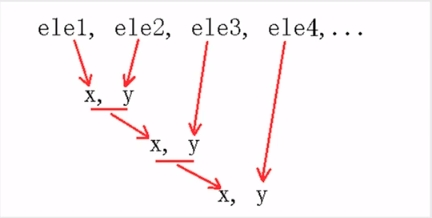• 定义一个求n阶乘函数 #include<stdio.h> int sum(int a,int x) { scanf("%d",&a); x=x*a; a=a-1; while(a>0) { x=x*a; a=a-1; } return x; } //以上为定义一个求a的阶乘的函数值为x int ...
定义一个求n的阶乘的函数
#include<stdio.h>
int sum(int a,int x)
{
x=1;
scanf("%d",&a);
x=x*a;
a=a-1;
while(a>0)
{
x=x*a;
a=a-1;
}
return x;
}          //这里是定义求a的阶乘的函数，a！=x
int main()
{
int n,m,d;
printf("请输入:");
if(n==0)
printf("1");   //0的阶乘是1
else
{
d=sum(n,m);
printf("%d\n",d);
}
return 0;
}


假设输入5，则结果如下图#include<stdio.h>
int main()
{
int n,i,x=1;
scanf("%d",&n);
for(i=n;i>=1;i--)    //i递减
{
x=x*n;
n=n-1;
}
printf("%d\n",x);
return 0;
}

#include<stdio.h>
int main()
{
int n,i,x=1;
scanf("%d",&n);
for(i=n;i>=1;i--)   //i递减
{
x=x*i;
//	  n=n-1;
}
printf("%d\n",x);
return 0;
}

#include<stdio.h>
int main()
{
int a=1,i,n;
scanf("%d",&n);
for(i=1;i<=n;i++)   //i递加
a=a*i;
printf("%d\n",a);
return 0;
}

假设输入5，则结果如下图递归方法做法如下
#include<stdio.h>
int sss(int a)
{
int b;
if(a==0||a==1)
b=1;
else
b=sss(a-1)*a;
return b;
}
int main()
{
int m,n;
scanf("%d",&m);
n=sss(m);
printf("%d\n",n);
}

运行结果如下展开全文• 定义一个求阶乘函数定义一个拥有6个元素的数组，调用求阶乘函数把1-6的阶乘分别放到数组的每一个元素中，就是a=1!,a=2!......a=6!,在此基础上求不是素数的和sum。 #include<stdio.h> main() { ...
定义一个求阶乘的函数，定义一个拥有6个元素的数组，调用求阶乘函数把1-6的阶乘分别放到数组的每一个元素中，就是a=1!,a=2!......a=6!，在此基础上求出数组元素的和sum。
#include<stdio.h>
main()
{
int fac(int n);
int a,i,j;
int sum=0;
for(i=0;i<6;i++)
a[i]=fac(i+1);
for(i=0;i<6;i++)
sum=sum+a[i];
printf("%d\n",sum);
for(i=0;i<6;i++)
printf("%d\t",a[i]);
}

int fac(int n)
{
int f;
if(n==0 || n==1)
f=1;
else
{
f=fac(n-1)*n;
return f;
}
}



展开全文c语言
• ## 递归函数求n的阶乘

万次阅读 2015-12-27 16:54:46
输入一个正整数n，利用递归函数求n阶乘。 递归函数声明如下： int fac(int n);  //求n!的递归函数声明 Input 一个正整数n Output n阶乘值 Sample Input 5 Sample Output 120 #include   ...

Description

输入一个正整数n，利用递归函数求n的阶乘。
递归函数声明如下：
int  fac(int n);  //求n!的递归函数声明

Input

一个正整数n

Output

n的阶乘值

Sample Input
5

Sample Output
120

#include <stdio.h>  #include <stdlib.h>      int main()  {      int fac(int n);      int n,y;      scanf("%d",&n);      y=fac(n);      printf("%d",y);      return 0;  }  int fac(int n)  {      int f;      if(n==0||n==1)f=1;      else f=fac(n-1)*n;      return (f);  }


展开全文• a = int(input("请输入一个数，计算其阶乘\n")) _sum = 1 for i in range(1,a+1): _sum *= i print(_sum) 使用递归 递归函数的使用一定要注意循环停止条件，这也是递归函数的难点。 # ...
Python案例实操
多种方式计算阶乘
循环
使用循环是最简单的一种方式，也比较实用。
# 方法一：使用循环来计算阶乘

a = int(input("请输入一个数，计算其阶乘\n"))

_sum = 1
for i in range(1,a+1):
_sum *= i

print(_sum)

使用递归
递归函数的使用一定要注意循环停止条件，这也是递归函数的难点。
# 方法二：使用递归来计算阶乘

a = int(input("请输入一个数，计算其阶乘\n"))

def fun(n):
if n == 1:
return 1
elif n == 0:
return 0
else:
return fun(n-1) * n

_sum = fun(a)
print(_sum)

使用reduce函数
Python在functools模块中提供了reduce()函数，该函数使用制定函数对序列对象进行累积可用过help(functools.reduce)来查看该函数的用法：reduce(function, sequence[, initial])
import functools

def fun(x,y):
return x*y

def fract(n):
if(n < 1):
return
else:
return functools.reduce(fun, range(1, n+1))
#   return functools.reduce(lambda x,y: x * y, range(1,n+1))
print(fract(5))

其中倒数第二、三行的作用相同，只不过倒数第二行更加简洁。reduce在本程序中作用就是从1到n，这些整数一个一个都分别给fun函数运行，达到了阶乘的目的。
展开全文reduce
• 问题及代码： 2717: 递归函数求n阶乘 Time Limit: 1 Sec Memory Limit: 128 MB Submit: 1177 Solved: 846 ...输入一个正整数n，利用递归函数求n阶乘。 递归函数声明如下： int fac(int n)
• <!DOCTYPE html> <title></title> //求阶乘n! 即，1×2×3×4×5×······×n（使用递归
• 定义n阶乘函数： 代码如下： def fact(n): s=1 for i in range(1,n+1): s*=i returnpython
• #define _CRT_SECURE_NO_WARNINGS #include&lt;stdio.h&gt; #include&lt;stdlib.h&...如果大于1，连续调用函数求阶乘 int ret = 1; if (a &gt; 1) { ret = a * fact(a...
• #include <iostream> using namespace std; int main () { int fac(int );//函数声明 int n,t; cin >...n;... t=fac (n);//函数调用 ...n<...int fac(int n)//函数定义 { int t; if(n==1||nc++ 递归算法
• 定义计算N阶乘函数 1）使用循环计算阶乘 def frac(n): r = 1 if n<=1: if n==0 or n==1: return 1 else: print('n 不能小于0') else: for i in range(1, n+1): ...python
• 思路：使用判断语句进行，使用一个变量作为控制。当其小于输入值时，另一个变量进行叠加。最终输出总和。 number=eval(input("请输入数字：")) def fact(n): i=1 s=0 while i<=number: s=s+i i+=1 return s...python
• 定义阶乘函数,再定义阶乘求和函数 #先定义阶乘函数 def jiecheng(n): ji = 1 for x in range(1,n+1): ji *= x return ji # 再定义阶乘求和函数 def jiechenghe(n): res = 0 for x in range(1,n+1): res +=...
• 主要介绍了PHP基于简单递归函数求一个阶乘的方法,结合实例形式分析了php递归函数定义与数学运算简单操作技巧,需要的朋友可以参考下
• #include using namespace std; long int func1(int n); int main() { int n,y; cin>>n; y=func1(n); cout; return 0; } long int func1(int n) { long int f;
• 编写递归函数int Fac(int n)来求n阶乘，然后在主函数中会调用该递归函数来计算S = M! + N!（M <= 10，N <= 10）的值。 所谓阶乘，是指从1乘以2乘以3乘以4一直乘到所要求的数。阶乘使用符号“!”来表示，如5...c++ c语言 c#
• #include <stdio.h> #include <...int factor(int n) {  int ret = 1;  int i = 1;  for (; i <= n; ++i)  {   ret *= i;  }  return ret; } int ma...
• ## 使用函数求阶乘和

千次阅读 2019-11-16 17:16:51
本题要求实现一个计算非负整数阶乘的简单函数，使得可以利用该函数1!+2!+…+n!的值。题目保证计算结果不超过双精度范围。 函数接口定义： double fact( int n ); 其中n是用户传入的参数。如果n是非负整数，则该...
• 方法 1.使用循环计算阶乘 2.运用递归计算阶乘 ...让循环计数器与前一个结果相乘，直到循环计数器等于N就得到N阶乘 #循环阶乘 def fract (n): r = 1 if n < 1: print('n不能小于1') return else: #...python
• 种： function jc() { if (n = 1) { return 1; } return n * jc(n - 1); }javascript
• 小例子:求n阶乘！ #include&amp;lt;stdio.h&amp;gt; int fun(int a) { if(a&amp;lt;=1) return 1; return(a*fun(a-1)); } int main() { int a,b; printf(&quot;input a num:\...
• 一个函数函数体中调用它本身。 2、示例 #include<stdio.h> int fac(int n) { int result; if(n<2) result = 1; else result = fac(n-1)*n; return result; } int main (int argc,intargv[]) { ...c语言
• 一个158位的整数100!这么大的数到底怎么算出来的呢？阶乘的计算直接求阶乘，需要经过大量的乘法运算，位数太多，计算机也无法表示出来。此时，往往采用对数方法，将阶乘的乘法运算化为加法运算。如编写一段Python...
• #include &amp;lt;stdio.h&amp;gt; #include &...写一个函数:x的阶乘 int getFactorial(int n) { int sum=1; int i; for (i=1;i&amp;lt;=n;i++) { sum*=i; } return sum; } int main () { int
• 广告关闭腾讯云11.11云上盛惠 ，精选热门产品助力上云，云服务器首年88元起，买的越多返的越多，最高返5000元！... 让阶乘编程一个简单的问题 解决方案我们可以先根据阶乘定义以及性质写出这样一个简...
• 广告关闭腾讯云11.11云上盛惠 ，精选热门产品助力上云，云服务器首年88元起，买的越多返的越多，最高返5000元！... 让阶乘编程一个简单的问题 解决方案我们可以先根据阶乘定义以及性质写出这样一个简......